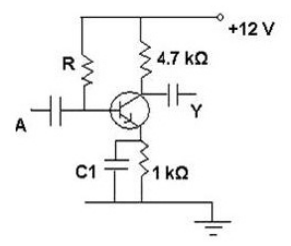Request a call back

If the common emitter current gain β of the transistor used in the amplifier circuit shown is 200, What will the quiescent base current be equal to if the quiescent emitter current is 1 mA.Asked by Topperlearning User | 10 Jul, 2014, 02:06: PMExpert Answer

In the common emitter mode, the current amplification factor (current gain) under no signal condition (βdc) is given by

βdc = IC/IB where IC is the collector current and IB is the base current (both under no signal conditions).

Since the collector current is almost equal to the emitter current IE (because of large value of βdc), we have

βdc ≈ IE/IB

Therefore IB ≈ IE/βdc =1 mA/200 = 0.005 mA = 5μA

Answered by | 10 Jul, 2014, 04:06: PM